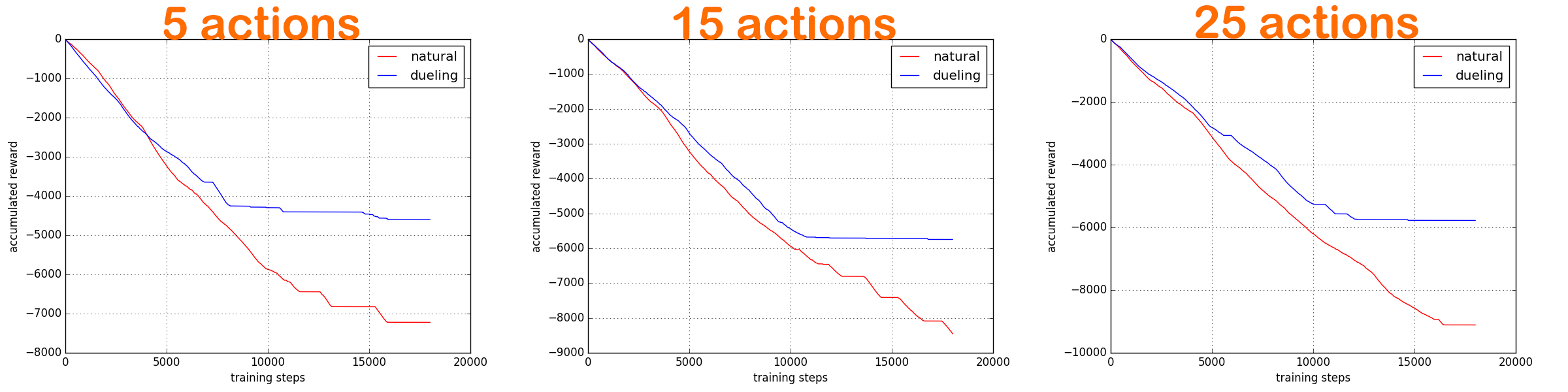# Dueling DQN (Tensorflow)

## Dueling 算法 ¶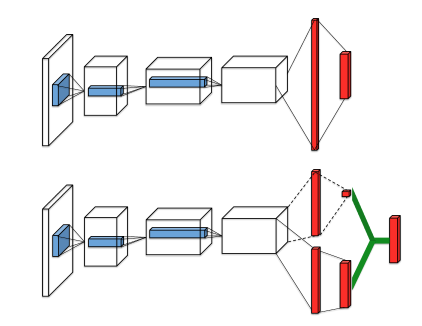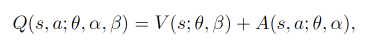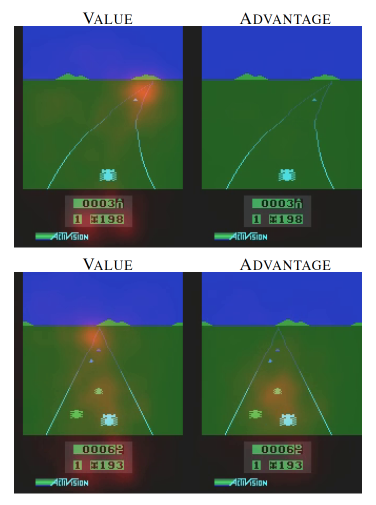## 更新方法 ¶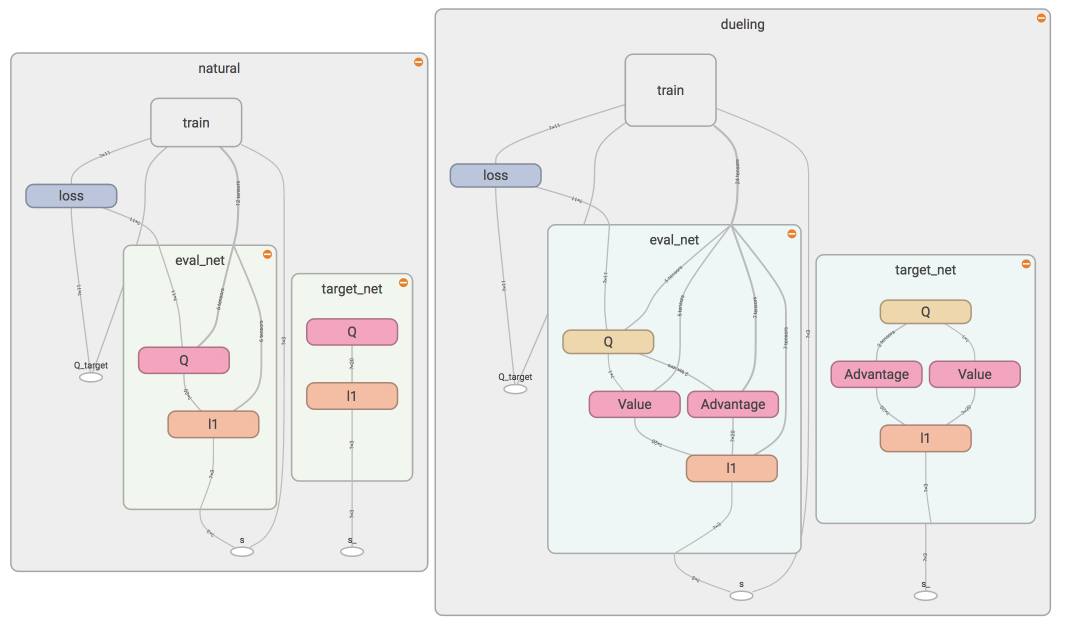class DuelingDQN:
def __init__(..., dueling=True, sess=None)
...
self.dueling = dueling  # 会建立两个 DQN, 其中一个是 Dueling DQN
...
if sess is None:    # 针对建立两个 DQN 的模式修改了 tf.Session() 的建立方式
self.sess = tf.Session()
self.sess.run(tf.global_variables_initializer())
else:
self.sess = sess
...

def _build_net(self):
def build_layers(s, c_names, n_l1, w_initializer, b_initializer):
with tf.variable_scope('l1'):   # 第一层, 两种 DQN 都一样
w1 = tf.get_variable('w1', [self.n_features, n_l1], initializer=w_initializer, collections=c_names)
b1 = tf.get_variable('b1', [1, n_l1], initializer=b_initializer, collections=c_names)
l1 = tf.nn.relu(tf.matmul(s, w1) + b1)

if self.dueling:
# Dueling DQN
with tf.variable_scope('Value'):    # 专门分析 state 的 Value
w2 = tf.get_variable('w2', [n_l1, 1], initializer=w_initializer, collections=c_names)
b2 = tf.get_variable('b2', [1, 1], initializer=b_initializer, collections=c_names)
self.V = tf.matmul(l1, w2) + b2

w2 = tf.get_variable('w2', [n_l1, self.n_actions], initializer=w_initializer, collections=c_names)
b2 = tf.get_variable('b2', [1, self.n_actions], initializer=b_initializer, collections=c_names)
self.A = tf.matmul(l1, w2) + b2

with tf.variable_scope('Q'):    # 合并 V 和 A, 为了不让 A 直接学成了 Q, 我们减掉了 A 的均值
out = self.V + (self.A - tf.reduce_mean(self.A, axis=1, keep_dims=True))     # Q = V(s) + A(s,a)
else:
with tf.variable_scope('Q'):    # 普通的 DQN 第二层
w2 = tf.get_variable('w2', [n_l1, self.n_actions], initializer=w_initializer, collections=c_names)
b2 = tf.get_variable('b2', [1, self.n_actions], initializer=b_initializer, collections=c_names)
out = tf.matmul(l1, w2) + b2

return out
...


## 对比结果 ¶